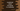# JavaScript program to check if an array is a subarray of another array## JavaScript program to check if an array is a subarray of another array:

In this post, we will learn different ways to check if an array is a subarray or not of another array in JavaScript. For example, [1, 2, 3] is a subarray of [1, 2, 3, 4, 5]. But [2, 12] is not a subarray of [1, 2, 3, 4, 5].

We can use two loops to check for each number of an array if that exists in an array or not. But, JavaScript provides other functions to do this without using any loop.

## Method 1: By using every:

every function takes one callback function as the argument and uses that function with all elements of the array. If this function returns true for each element of the array, every() will return true.

We can use includes() function to check if the current element iterating by every() is in the second array or not.

Complete program:

``````let firstArray = [1, 2, 3, 4, 5, 6];
let secondArray = [2, 3, 5];

let isSubArr = secondArray.every(e => firstArray.includes(e));

if(isSubArr)
console.log('secondArray is a subarray of firstArray')
else
console.log('secondArray is not a subarray of firstArray')``````
• It is checking if secondArray is a subarray of firstArray or not.
• We are calling every on secondArray, i.e. it checks for every element of secondArray. The arrow function checks if every element is in firstArray or not.

If you run the above program, it will print secondArray is a subarray of firstArray. You can also try it with different arrays to see how it behaves.

### every and indexof:

We can also use indexOf instead of includes. indexOf will return -1 if the element is not found in the array. Else, it returns the index of that element.

``````let firstArray = [1, 2, 3, 4, 5, 6];
let secondArray = [2, 3, 5];

let isSubArr = secondArray.every(e => firstArray.indexOf(e) !== -1);

if(isSubArr)
console.log('secondArray is a subarray of firstArray')
else
console.log('secondArray is not a subarray of firstArray')``````

It will print a similar output.

## Method 2: Using some():

some checks if some element satisfies a condition. We can check if there are some elements, which are not in the original array and we can reverse the result. some returns one boolean value. It takes one callback function similar to every.

We can use indexOf or includes with some() to get the result.

``````let firstArray = [1, 2, 3, 4, 5, 6];
let secondArray = [2, 3, 5];

let isSubArr = !secondArray.some(e => !firstArray.includes(e));

if(isSubArr)
console.log('secondArray is a subarray of firstArray')
else
console.log('secondArray is not a subarray of firstArray')``````

It will give similar result as the above examples.

We can also use indexOf with some():

``````let firstArray = [1, 2, 3, 4, 5, 6];
let secondArray = [2, 3, 5];

let isSubArr = !secondArray.some(e => firstArray.indexOf(e) == -1);

if(isSubArr)
console.log('secondArray is a subarray of firstArray')
else
console.log('secondArray is not a subarray of firstArray')``````

### Method 3: By creating a Set:

Another way is to create a new Set with the contents of both sets. A Set can hold only unique values.

If secondArray is a subarray of firstArray, the length of the set will be equal to firstArray, because each element of secondArray is in firstArray and a set can’t have duplicate values.

Program:

``````let firstArray = [1, 2, 3, 4, 5, 6];
let secondArray = [2, 3, 5];

let isSubArr = new Set([...firstArray, ...secondArray]).size === firstArray.length;

if(isSubArr)
console.log('secondArray is a subarray of firstArray')
else
console.log('secondArray is not a subarray of firstArray')``````

It will print similar output.